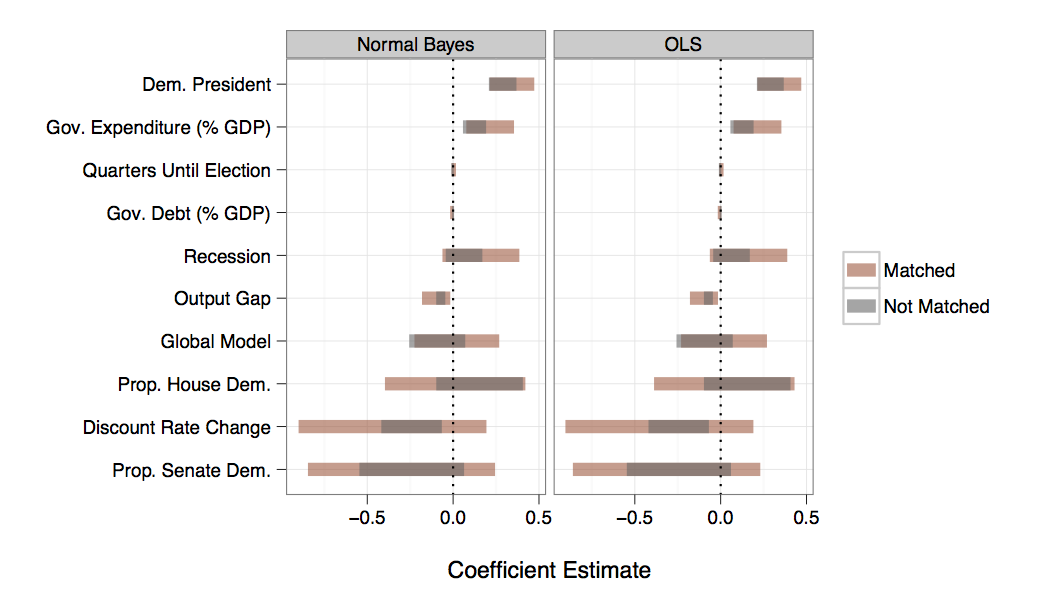### Graphically Comparing Confidence Intervals From Different Models

In a recent paper on Federal Reserve inflation forecast errors (summary blog post, paper) I wanted a way to easily compare the coefficients for a set of covariates (a) estimated from different types of parametric models using (b) matched and non-matched data.

I guess the most basic way to do this would be to have a table of columns showing point estimates and confidence intervals from each estimation model. But making meaningful comparisons with this type of table would be tedious.

What I ended up doing was creating a kind of stacked caterpillar plot. Here it is:I think this plot lets you clearly and quickly compare the confidence intervals estimated from the different models. I didn't include the coefficient point estimates because I was most interested in comparing the ranges. The dots added too much clutter.

I have a link to the full replication code at the end of the post, but these are the basic steps:

1. I estimated the models using MatchIt and Zelig as per Ho et al. (2007). I created new objects from the results of each model.

2. I used the `confint` command to find the 95% confidence intervals.

3. I did some cleaning up and rearranging of the confidence intervals, mostly using Hadley Wickham's `melt` function in the reshape package. The basic idea is that to create the plots I needed a data set with columns for the coefficient names, the upper and lower confidence interval bounds, what parametric model the estimates are from, and whether the data set was matched or not. I removed the `Intercept` and `sigma2` estimates for simplicity.

4. I made the graph using ggplot2. The key aesthetic decisions that I think make it easier to read are: (a) making the lines a bit thicker and (b) making the bands transparent. I liked making the bands transparent and stacking them rather than showing different lines for each set of estimates because this halved the number of lines in the plot. Makes it much crisper.

The full code for replicating this figure is on GitHub Note: this code depends on objects that are created as the result of analyses run using other source code files also on the GitHub site.

### Showing results from Cox Proportional Hazard Models in R with simPH

Update 2 February 2014: A new version of simPH (Version 1.0) will soon be available for download from CRAN. It allows you to plot using points, ribbons, and (new) lines. See the updated package description paper for examples. Note that the ribbons argument will no longer work as in the examples below. Please use type = 'ribbons' (or 'points' or 'lines' ). Effectively showing estimates and uncertainty from Cox Proportional Hazard (PH) models , especially for interactive and non-linear effects, can be challenging with currently available software. So, researchers often just simply display a results table. These are pretty useless for Cox PH models. It is difficult to decipher a simple linear variable’s estimated effect and basically impossible to understand time interactions, interactions between variables, and nonlinear effects without the reader further calculating quantities of interest for a variety of fitted values. So, I’ve been putting together th

### Slide: one function for lag/lead variables in data frames, including time-series cross-sectional data

I often want to quickly create a lag or lead variable in an R data frame. Sometimes I also want to create the lag or lead variable for different groups in a data frame, for example, if I want to lag GDP for each country in a data frame. I've found the various R methods for doing this hard to remember and usually need to look at old blog posts . Any time we find ourselves using the same series of codes over and over, it's probably time to put them into a function. So, I added a new command– slide –to the DataCombine R package (v0.1.5). Building on the shift function TszKin Julian posted on his blog , slide allows you to slide a variable up by any time unit to create a lead or down to create a lag. It returns the lag/lead variable to a new column in your data frame. It works with both data that has one observed unit and with time-series cross-sectional data. Note: your data needs to be in ascending time order with equally spaced time increments. For example 1995, 1996